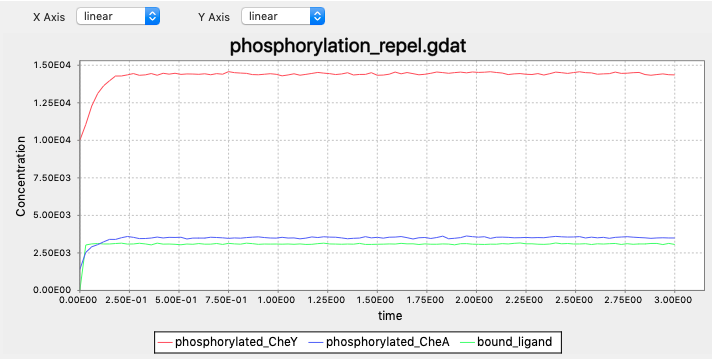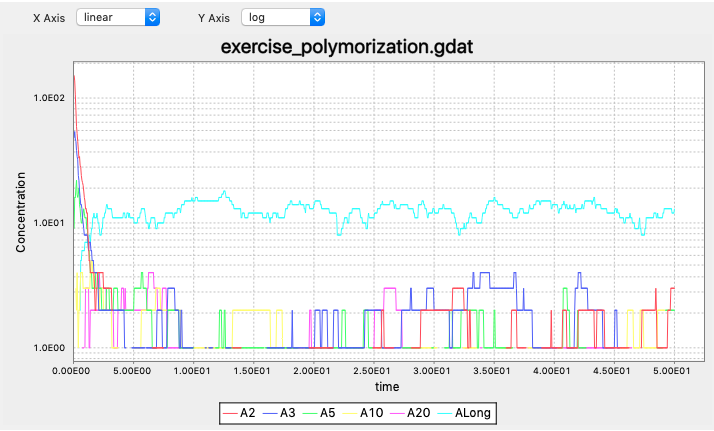# Solutions

## How do E. coli respond to repellents?

Exercise 1

In contrast to that CheY phosphorylations decrease and tumbling becomes less frequent when the cell senses higher attractant concentrations, when the cell senses more repellents there should be more frequent tumbling. The decreased tumbling frequency should be a result of increased CheY phosphorylations. The cell should always be able to adapt to the current concentrations, therefore we also expect the CheY phosphoryaltions be restored when adpating.

Exercise 2

Update reaction rule for ligand-receptor binding from

BoundTP: L(t!1).T(l!1,Phos~U) -> L(t!1).T(l!1,Phos~P) k_T_phos*0.2


to

BoundTP: L(t!1).T(l!1,Phos~U) -> L(t!1).T(l!1,Phos~P) k_T_phos*5


The complete code (you can download a completed BioNetGen file here: exercise_repel.bngl):

begin model

begin molecule types
L(t)             #ligand molecule
T(l,Phos~U~P)    #receptor complex
CheY(Phos~U~P)
CheZ()
end molecule types

begin parameters
NaV2 6.02e8   #Unit conversion to cellular concentration M/L -> #/um^3
L0 5e3          #number of ligand molecules
T0 7000       #number of receptor complexes
CheY0 20000
CheZ0 6000

k_lr_bind 8.8e6/NaV2   #ligand-receptor binding
k_lr_dis 35            #ligand-receptor dissociation
k_T_phos 15            #receptor complex autophosphorylation
k_Y_phos 3.8e6/NaV2    #receptor complex phosphorylates Y
k_Y_dephos 8.6e5/NaV2  #Z dephosphorylates Y
end parameters

begin reaction rules
LR: L(t) + T(l) <-> L(t!1).T(l!1) k_lr_bind, k_lr_dis

#Free vs. ligand-bound receptor complexes autophosphorylates at different rates
FreeTP: T(l,Phos~U) -> T(l,Phos~P) k_T_phos
BoundTP: L(t!1).T(l!1,Phos~U) -> L(t!1).T(l!1,Phos~P) k_T_phos*5

YP: T(Phos~P) + CheY(Phos~U) -> T(Phos~U) + CheY(Phos~P) k_Y_phos
YDeps: CheZ() + CheY(Phos~P) -> CheZ() + CheY(Phos~U) k_Y_dephos
end reaction rules

begin species
L(t) L0
T(l,Phos~U) T0*0.8
T(l,Phos~P) T0*0.2
CheY(Phos~U) CheY0*0.5
CheY(Phos~P) CheY0*0.5
CheZ() CheZ0
end species

begin observables
Molecules phosphorylated_CheY CheY(Phos~P)
Molecules phosphorylated_CheA T(Phos~P)
Molecules bound_ligand L(t!1).T(l!1)
end observables

end model

generate_network({overwrite=>1})
simulate({method=>"ssa", t_end=>3, n_steps=>100})


The simulation outputs:## What if there are multiple attractant sources?

Exercise 1:

In molecule types and observables, update L(t) and T(l,r,Meth~A~B~C,Phos~U~P) to L(t,Lig~A~B) and T(l,r,Lig~A~B,Meth~A~B~C,Phos~U~P), where A and B represent the two ligand types. Update the reaction rule

LR: L(t) + T(l) <-> L(t!1).T(l!1) k_lr_bind, k_lr_dis


to

L1R: L(t,Lig~A) + T(l,Lig~A) <-> L(t!1,Lig~A).T(l!1,Lig~A) k_lr_bind, k_lr_dis
L2R: L(t,Lig~B) + T(l,Lig~B) <-> L(t!1,Lig~B).T(l!1,Lig~B) k_lr_bind, k_lr_dis


Also update the species by equally split the initial receptor concentrations by 2.

Exercise 2:

To wait for adaptation to ligand A, we could replace the forward reaction rate with this rule: rate constant = 0 unless after adapting to A. We could run the simulation without B first and observe the equilibrium methylation states, and use this for deciding whether the cell is adapted to A. (Why not equilibrium concentrations of free A?) One possible implementation is the following: replace

L1R: L(t,Lig~A) + T(l,Lig~A) <-> L(t!1,Lig~A).T(l!1,Lig~A) k_lr_bind, k_lr_dis
L2R: L(t,Lig~B) + T(l,Lig~B) <-> L(t!1,Lig~B).T(l!1,Lig~B) k_lr_bind, k_lr_dis


with

L1R: L(t,Lig~A) + T(l,Lig~A) <-> L(t!1,Lig~A).T(l!1,Lig~A) k_lr_bind, k_lr_dis
L2R: L(t,Lig~B) + T(l,Lig~B) <-> L(t!1,Lig~B).T(l!1,Lig~B) l2rate(), k_lr_dis


and l2rate() is a function defined as (remember to define it before reaction rules)

begin functions
l2rate() = if(high_methyl_receptor>1.2e3,k_lr_bind,0)
end functions


The complete code:

begin model

begin compartments
EC  3  100       #um^3
PM  2  1   EC    #um^2
CP  3  1   PM    #um^3
end compartments

begin molecule types
L(t,Lig~A~B)
T(l,r,Lig~A~B,Meth~A~B~C,Phos~U~P)
CheY(Phos~U~P)
CheZ()
CheB(Phos~U~P)
CheR(t)
end molecule types

begin observables
Molecules bound_ligand L(t!1).T(l!1)
Molecules phosphorylated_CheY CheY(Phos~P)
Molecules low_methyl_receptor T(Meth~A)
Molecules medium_methyl_receptor T(Meth~B)
Molecules high_methyl_receptor T(Meth~C)
Molecules phosphorylated_CheB CheB(Phos~P)
end observables

begin parameters
NaV2 6.02e8   #Unit conversion to cellular concentration M/L -> #/um^3
miu 1e-6

L0 1e6
T0 7000
CheY0 20000
CheZ0 6000
CheR0 120
CheB0 250

k_lr_bind 8.8e6/NaV2   #ligand-receptor binding
k_lr_dis 35            #ligand-receptor dissociation

k_TaUnbound_phos 7.5   #receptor complex autophosphorylation

k_Y_phos 3.8e6/NaV2    #receptor complex phosphorylates Y
k_Y_dephos 8.6e5/NaV2  #Z dephosphorylates Y

k_TR_bind 2e7/NaV2          #Receptor-CheR binding
k_TR_dis  1            #Receptor-CheR dissociaton
k_TaR_meth 0.08        #CheR methylates receptor complex

k_B_phos 1e5/NaV2      #CheB phosphorylation by receptor complex
k_B_dephos 0.17        #CheB autodephosphorylation

k_Tb_demeth 5e4/NaV2   #CheB demethylates receptor complex
k_Tc_demeth 2e4/NaV2   #CheB demethylates receptor complex
end parameters

begin functions
l2rate() = if(high_methyl_receptor>1.2e3,k_lr_bind,0)
end functions

begin reaction rules
L1R: L(t,Lig~A) + T(l,Lig~A) <-> L(t!1,Lig~A).T(l!1,Lig~A) k_lr_bind, k_lr_dis
L2R: L(t,Lig~B) + T(l,Lig~B) <-> L(t!1,Lig~B).T(l!1,Lig~B) l2rate(), k_lr_dis
#L3R: L(t,Lig~T) + T(l,Lig~O) <-> L(t!1,Lig~O).T(l!1,Lig~O) l2rate(), k_lr_dis

#Receptor complex (specifically CheA) autophosphorylation
#Rate dependent on methylation and binding states
#Also on free vs. bound with ligand
TaUnboundP: T(l,Meth~A,Phos~U) -> T(l,Meth~A,Phos~P) k_TaUnbound_phos
TbUnboundP: T(l,Meth~B,Phos~U) -> T(l,Meth~B,Phos~P) k_TaUnbound_phos*1.1
TcUnboundP: T(l,Meth~C,Phos~U) -> T(l,Meth~C,Phos~P) k_TaUnbound_phos*2.8
TaLigandP: L(t!1).T(l!1,Meth~A,Phos~U) -> L(t!1).T(l!1,Meth~A,Phos~P) 0
TbLigandP: L(t!1).T(l!1,Meth~B,Phos~U) -> L(t!1).T(l!1,Meth~B,Phos~P) k_TaUnbound_phos*0.8
TcLigandP: L(t!1).T(l!1,Meth~C,Phos~U) -> L(t!1).T(l!1,Meth~C,Phos~P) k_TaUnbound_phos*1.6

#CheY phosphorylation by T and dephosphorylation by CheZ
YP: T(Phos~P) + CheY(Phos~U) -> T(Phos~U) + CheY(Phos~P) k_Y_phos
YDep: CheZ() + CheY(Phos~P) -> CheZ() + CheY(Phos~U) k_Y_dephos

#CheR binds to and methylates receptor complex
#Rate dependent on methylation states and ligand binding
TRBind: T(r) + CheR(t) <-> T(r!2).CheR(t!2) k_TR_bind, k_TR_dis
TaRUnboundMeth: T(r!2,l,Meth~A).CheR(t!2) -> T(r,l,Meth~B) + CheR(t) k_TaR_meth
TbRUnboundMeth: T(r!2,l,Meth~B).CheR(t!2) -> T(r,l,Meth~C) + CheR(t) k_TaR_meth*0.1
TaRLigandMeth: T(r!2,l!1,Meth~A).L(t!1).CheR(t!2) -> T(r,l!1,Meth~B).L(t!1) + CheR(t) k_TaR_meth*30
TbRLigandMeth: T(r!2,l!1,Meth~B).L(t!1).CheR(t!2) -> T(r,l!1,Meth~C).L(t!1) + CheR(t) k_TaR_meth*3

#CheB is phosphorylated by receptor complex, and autodephosphorylates
CheBphos: T(Phos~P) + CheB(Phos~U) -> T(Phos~U) + CheB(Phos~P) k_B_phos
CheBdephos: CheB(Phos~P) -> CheB(Phos~U) k_B_dephos

#CheB demethylates receptor complex
#Rate dependent on methyaltion states
TbDemeth: T(Meth~B) + CheB(Phos~P) -> T(Meth~A) + CheB(Phos~P) k_Tb_demeth
TcDemeth: T(Meth~C) + CheB(Phos~P) -> T(Meth~B) + CheB(Phos~P) k_Tc_demeth

end reaction rules

begin species
@EC:L(t,Lig~A) L0
@EC:L(t,Lig~B) L0
@PM:T(l,r,Lig~A,Meth~A,Phos~U) T0*0.84*0.9*0.5
@PM:T(l,r,Lig~A,Meth~B,Phos~U) T0*0.15*0.9*0.5
@PM:T(l,r,Lig~A,Meth~C,Phos~U) T0*0.01*0.9*0.5
@PM:T(l,r,Lig~A,Meth~A,Phos~P) T0*0.84*0.1*0.5
@PM:T(l,r,Lig~A,Meth~B,Phos~P) T0*0.15*0.1*0.5
@PM:T(l,r,Lig~A,Meth~C,Phos~P) T0*0.01*0.1*0.5
@PM:T(l,r,Lig~B,Meth~A,Phos~U) T0*0.84*0.9*0.5
@PM:T(l,r,Lig~B,Meth~B,Phos~U) T0*0.15*0.9*0.5
@PM:T(l,r,Lig~B,Meth~C,Phos~U) T0*0.01*0.9*0.5
@PM:T(l,r,Lig~B,Meth~A,Phos~P) T0*0.84*0.1*0.5
@PM:T(l,r,Lig~B,Meth~B,Phos~P) T0*0.15*0.1*0.5
@PM:T(l,r,Lig~B,Meth~C,Phos~P) T0*0.01*0.1*0.5
@CP:CheY(Phos~U) CheY0*0.71
@CP:CheY(Phos~P) CheY0*0.29
@CP:CheZ() CheZ0
@CP:CheB(Phos~U) CheB0*0.62
@CP:CheB(Phos~P) CheB0*0.38
@CP:CheR(t) CheR0
end species

end model

generate_network({overwrite=>1})
simulate({method=>"ssa", t_end=>700, n_steps=>400})


Exercise 3:

Define ligand_center1 = [1500, 1500] and ligand_center2 = [-1500, 1500]. Since we are considering two gradients, we can add up the ligand concentration. We can replace our cal_concentraion(pos) function with

def calc_concentration(pos):
dist1 = euclidean_distance(pos, ligand_center1)
dist2 = euclidean_distance(pos, ligand_center2)

exponent1 = (1 - dist1 / origin_to_center) * (center_exponent - start_exponent) + start_exponent
exponent2 = (1 - dist2 / origin_to_center) * (center_exponent - start_exponent) + start_exponent

return 10 ** exponent1 + 10 ** exponent2


## Is the actual tumbling reorientation used by E. coli smarter than our model?

Now, for sampling the new direction, we need to consider the past concentration and the current concentration the bacterium experiences. Since the new direction is also dependent on the last direction, we also need to record the current directions.

Therefore, for our tumble_move() function, we would consider three inputs: curr_direction, curr_conc, past_conc. If the current concentration is higher than the past concentration, we sample the turning with mean of 1.19π-0.1π=1.09π and standard deviation of 0.63π; otherwise ample the turning with mean of 1.19π and standard deviation of 0.63π. The new direction is the sum of the turning and the past direction.

Add the mean and standard deviation of turning as constants.

#Constants for E.coli tumbling
tumble_angle_mu = 1.19
tumble_angle_std = 0.63


We implement the tumble_move function as the following:

def tumble_move(curr_dir, curr_conc, past_conc):
#Sample the new direction
corrent = curr_conc > past_conc

if correct:
new_dir = np.random.normal(loc = tumble_angle_mu - 0.1, scale = tumble_angle_std)
else:
new_dir = np.random.normal(loc = tumble_angle_mu, scale = tumble_angle_std)
new_dir *= np.random.choice([-1, 1])
new_dir += curr_dir

new_dir = new_dir % (2 * math.pi) #keep within [0, 2pi]

projection_h = math.cos(new_dir) #Horizontal displacement for next run
projection_v = math.sin(new_dir) #Vertical displacement for next run

tumble_time = np.random.exponential(tumble_time_mu) #Length of the tumbling

return new_dir, projection_h, projection_v, tumble_time


Update the simulate function by replacing

projection_h, projection_v, tumble_time = tumble_move()


with

curr_direction, projection_h, projection_v, tumble_time = tumble_move(curr_direction, curr_conc, past_conc)


## Can’t get enough BioNetGen?

Exercise 1: You should know the molecules involved (molecule types), reactions and reaction rate constants (reaction rules), the initial conditions (species), the quantities you are interested in observing (observables), your simulation methods and time steps. Compartments and parameters should also be considered if applicable.

Exercise 2: The complete code (you can download a completed BioNetGen file here: exercise_polymerization.bngl):

begin model

begin molecule types
A(h,t)
end molecule types

begin reaction rules
Initiation: A(h,t) + A(h,t) <-> A(h,t!1).A(h!1,t) 0.01,0.01
Polymerizationfree: A(h!+,t) + A(h,t) <-> A(h!+,t!1).A(h!1,t) 0.01,0.01
Polymerizationfree2: A(h,t) + A(h,t!+) <-> A(h,t!1).A(h!1,t!+) 0.01,0.01
Polymerizationbound: A(h!+,t) + A(h,t!+) <-> A(h!+,t!1).A(h!1,t!+) 0.01,0.01
end reaction rules

begin species
A(h,t) 1000
end species

begin observables
Species A1 A==1
Species A2 A==2
Species A3 A==3
Species A5 A==5
Species A10 A==10
Species A20 A==20
Species ALong A>=30
end observables

end model

simulate({method=>"nf", t_end=>50, n_steps=>1000})


The simulation outputs (note the concentrations are in log-scale):## How to calculate steady state concentration in a reversible bimolecular reaction?

Exercise 1: When the reaction begins, concentrations change toward the equilibrium concentrations. The system remains at the equilibrium state once reaching it.

Exercise 2: Use [A], [B], [AB] to denote the equilibrium concentrations. At equilibrium concentrations, we have

kbind · [A] · [B] = kdissociate · [AB].

Because of conservation of mass, if the instead starts from no AB, our initial conditions will be a0 = b0 = 100, and ab0 = 0. (If we instead work from the “current” concentrations, a0 = b0 = 95, and ab0 = 5, how would you set up the calculations?)

Similar as in the main text, Our original steady state equation can be modified to

kbind · (a0 - [AB]) · (b0 - [AB]) = kdissociate · [AB].

Solving this equation yields [AB] = 90.488.

Exercise 3: If we add additional 100 A molecules to the system, more AB will be formed. If you use the equation setup in the solution above, we can simply update a0 = 200. [AB] = 99.019.

If kdissociate = 9 instead of 3, less AB will be present at the equilibirum state. [AB] = 84.115.

## How to simulate a reaction step with the Gillespie algorithm?

Exercise 1: Shorter because molecules collide to each other and react more frequently.

Exercise 2: In this system, we have λ = 100. The probability that exactly 100 reaction happen in the next second is

$\mathrm{Pr}(X = 100) = \dfrac{\lambda^n e^{-\lambda}}{n!} = 0.03986\,.$

The expected wait time is 1/λ = 0.01.

The probability that the first reaction occur after 0.02 second is

$\mathrm{Pr}(T > 0.02) = e^{-\lambda t} = 0.1353\,.$

Exercise 3: At the beginning of the simulation, only one type of reaction could occur: L + TLT. The rate of reaction is kbind[L][T] = 100molecule·s-1. Therefore we have λ = 100molecule·s-1, and the expected wait time is thus 1/λ = 0.01s·molecule-1.

Although the expected wait time before the first reaction is considerably shorter than 0.1s, it is still possible for the first reaction to happen after 0.1s.

After the first reaction, our system has 9 L, 9 T, and 1 LT molecules. There are two possible types of reactions to occur: the forward reaction L + TLT and the reverse reaction LTL + T. The rate of forward reaction is kbind[L][T] = 81molecule·s-1, while the rate of reverse reaction is kdissociate[LT] = 2molecule·s-1. The total reaction rate is 83molecule·s-1 and hence the expected wait time before the next reaction is 0.012s. The probability of forward reaction is 81molecule·s-1/83molecule·s-1 = 0.976, and the probability of reverse reaction is 0.0241.